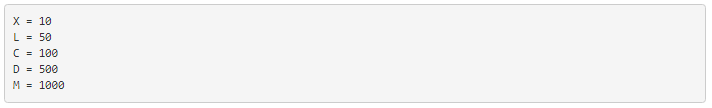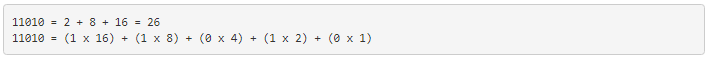# 1.2: Systems of Numeration

The Romans devised a system that was a substantial improvement over hash marks, because it used a variety of symbols (or ciphers) to represent increasingly large quantities. The notation for 1 is the capital letter I. The notation for 5 is the capital letter V. Other ciphers possess increasing values:If a cipher is accompanied by another cipher of equal or lesser value to the immediate right of it, with no ciphers greater than that other cipher to the right of that other cipher, that other cipher’s value is added to the total quantity. Thus, VIII symbolizes the number 8, and CLVII symbolizes the number 157. On the other hand, if a cipher is accompanied by another cipher of lesser value to the immediate left, that other cipher’s value is subtracted from the first. Therefore, IV symbolizes the number 4 (V minus I), and CMsymbolizes the number 900 (M minus C). You might have noticed that ending credit sequences for most motion pictures contain a notice for the date of production, in Roman numerals. For the year 1987, it would read: MCMLXXXVII. Let’s break this numeral down into its constituent parts, from left to right:Aren’t you glad we don’t use this system of numeration? Large numbers are very difficult to denote this way, and the left vs. right / subtraction vs. addition of values can be very confusing, too. Another major problem with this system is that there is no provision for representing the number zero or negative numbers, both very important concepts in mathematics. Roman culture, however, was more pragmatic with respect to mathematics than most, choosing only to develop their numeration system as far as it was necessary for use in daily life.

We owe one of the most important ideas in numeration to the ancient Babylonians, who were the first (as far as we know) to develop the concept of cipher position, or place value, in representing larger numbers. Instead of inventing new ciphers to represent larger numbers, as the Romans did, they re-used the same ciphers, placing them in different positions from right to left. Our own decimal numeration system uses this concept, with only ten ciphers (0, 1, 2, 3, 4, 5, 6, 7, 8, and 9) used in “weighted” positions to represent very large and very small numbers.

Each cipher represents an integer quantity, and each place from right to left in the notation represents a multiplying constant, or weight, for each integer quantity. For example, if we see the decimal notation “1206”, we known that this may be broken down into its constituent weight-products as such:Each cipher is called a digit in the decimal numeration system, and each weight, or place value, is ten times that of the one to the immediate right. So, we have a ones place, a tens place, a hundreds place, a thousands place, and so on, working from right to left.

Right about now, you’re probably wondering why I’m laboring to describe the obvious. Who needs to be told how decimal numeration works, after you’ve studied math as advanced as algebra and trigonometry? The reason is to better understand other numeration systems, by first knowing the how’s and why’s of the one you’re already used to.

The decimal numeration system uses ten ciphers, and place-weights that are multiples of ten. What if we made a numeration system with the same strategy of weighted places, except with fewer or more ciphers?

The binary numeration system is such a system. Instead of ten different cipher symbols, with each weight constant being ten times the one before it, we only have two cipher symbols, and each weight constant is twice as much as the one before it. The two allowable cipher symbols for the binary system of numeration are “1” and “0,” and these ciphers are arranged right-to-left in doubling values of weight. The rightmost place is the ones place, just as with decimal notation. Proceeding to the left, we have the twos place, the foursplace, the eights place, the sixteens place, and so on. For example, the following binary number can be expressed, just like the decimal number 1206, as a sum of each cipher value times its respective weight constant:This can get quite confusing, as I’ve written a number with binary numeration (11010), and then shown its place values and total in standard, decimal numeration form (16 + 8 + 2 = 26). In the above example, we’re mixing two different kinds of numerical notation. To avoid unnecessary confusion, we have to denote which form of numeration we’re using when we write (or type!). Typically, this is done in subscript form, with a “2” for binary and a “10” for decimal, so the binary number 110102 is equal to the decimal number 2610.

The subscripts are not mathematical operation symbols like superscripts (exponents) are. All they do is indicate what system of numeration we’re using when we write these symbols for other people to read. If you see “310”, all this means is the number three written using decimal numeration. However, if you see “310”, this means something completely different: three to the tenth power (59,049). As usual, if no subscript is shown, the cipher(s) are assumed to be representing a decimal number.

Commonly, the number of cipher types (and therefore, the place-value multiplier) used in a numeration system is called that system’s base. Binary is referred to as “base two” numeration, and decimal as “base ten.” Additionally, we refer to each cipher position in binary as a bit rather than the familiar word digit used in the decimal system.

Now, why would anyone use binary numeration? The decimal system, with its ten ciphers, makes a lot of sense, being that we have ten fingers on which to count between our two hands. (It is interesting that some ancient central American cultures used numeration systems with a base of twenty. Presumably, they used both fingers and toes to count!!). But the primary reason that the binary numeration system is used in modern electronic computers is because of the ease of representing two cipher states (0 and 1) electronically. With relatively simple circuitry, we can perform mathematical operations on binary numbers by representing each bit of the numbers by a circuit which is either on (current) or off (no current). Just like the abacus with each rod representing another decimal digit, we simply add more circuits to give us more bits to symbolize larger numbers. Binary numeration also lends itself well to the storage and retrieval of numerical information: on magnetic tape (spots of iron oxide on the tape either being magnetized for a binary “1” or demagnetized for a binary “0”), optical disks (a laser-burned pit in the aluminum foil representing a binary “1” and an unburned spot representing a binary “0”), or a variety of other media types.

Before we go on to learning exactly how all this is done in digital circuitry, we need to become more familiar with binary and other associated systems of numeration.